IF YOU CAN SEE THIS go to /sfa/Nuprl/Shared/Xindentation_hack_doc.html

### Reconciling Russell's "Orders" with Prop Levels

Type levels and propositional levels are explained in Universes and Prop Levels and Predicativity.

Bertrand Russell's "ramified" theory of types is the principal ancestor to intuitionistic type theory. Russell conceives of a type as a "range of significance" for a propositional function. In an impredicative logic, such as Frege's, the notion of type is "extensional" in that the type of a functional expression is determined wholly by its input and output types. For example, in Frege's logic, the types are basically these: individuals; one-place functions of individuals; one-place functions of one-place functions of individuals; two-place functions on individuals; two-place functions taking one individual and one one-place function of individuals; and so forth.

In contrast, in addition to this simple extensional type structure, there is an "intensional" aspect to Russell's types, hence the nomenclature "ramified" (meaning "branching"). Russell assigns "orders": individuals are the lowest order entities; then come propositions that don't involve quantification over propositions; then propositions that involve quantification over propositions of the previous sort; and so forth with various mixtures.

Here is Nuprl's approximation of order in ramified type theory.

In Nuprl's type theory the members of Type{1} are themselves types of individuals; call them first-order types. The members of Prop{1} are propositions involving at most quantification over first-order types of individuals; call them first-order propositions. The members of Type{2} may be totalities definable given all of Type{1} and Prop{1} themselves as classes of entities; call them second-order types (thus Type{1} and Prop{1} are second-order types). The members of Prop{2} are propositions involving at most quantification over second-order types including Type{1} and Prop{1}. And so forth.

Observe that we have included lower-order types among higher-order types, and lower-order propositions among higher-order propositions. This has a certain convenience, but is actually critical in intuitionistic computational type theory since one cannot effectively recognize when a second-order type or proposition, say, is actually first-order. For example,

Thm*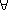f:(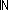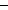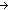). (n:. if f(n)=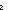0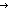False elseC:Prop{1}. C fi)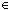Prop{2}

But whether (n:. if f(n)=0False ;C:Prop{1}. C fi)Prop{1} depends on whether f is everywhere zero, which is not in general recognizable.

Thm*f:().
Thm* (n:f(n) = 0)
Thm*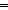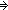Thm* (n:. if f(n)=0False elseC:Prop{1}. C fi)Prop{1}

(March 2001 - sfa )